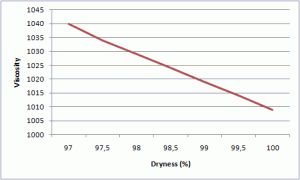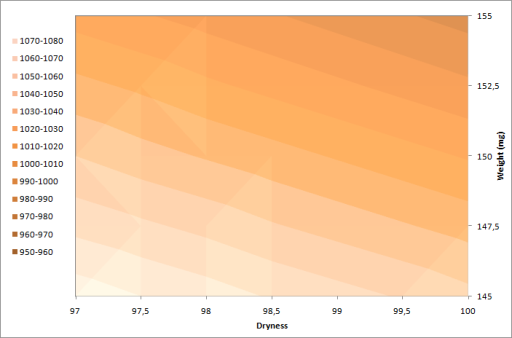# Dryness & Weight

### Two of the important parameter where reliable values are required are the sample dryness and the sample weight.

Some labs use fixed, estimated, dryness (e.g. 100%) instead of measuring the actual dryness of the sample. This is normally done in order to reduce the time for the analysis. Similarly, some labs use a fixed weight (e.g 150 mg) instead of the actual weight of the used sample. As can be seen from the discussion below, having the correct values for these two parameters are important.

The figure below shows the change in viscosity due to changing the dryness, created from recalculation of an analyzed sample. At a dryness value of 100%, the viscosity is 1008 and at 97% the viscosity is 1040, 3.2% higher.The next figure shows the change in viscosity due to changing the weight. By using a weight of 145 mg, the viscosity is 1054 and with 155 mg the viscosity is 986, 6.5% lower.Without having proper control of neither dryness or weight, the values might deviate significantly, as can be seen from the figure below.